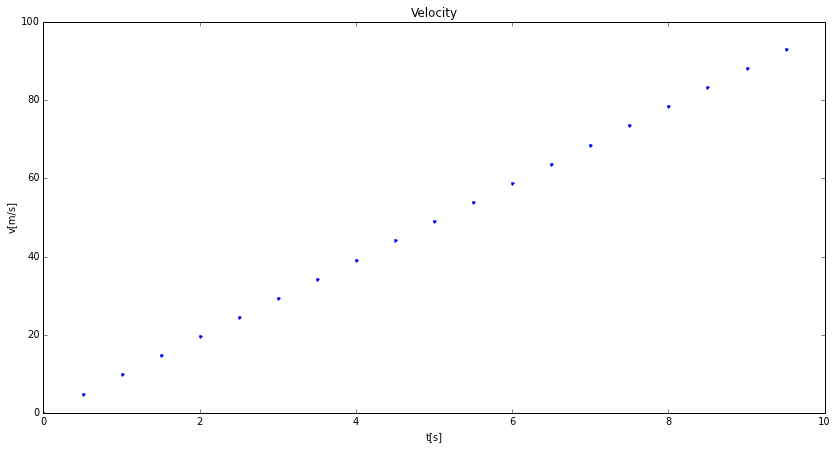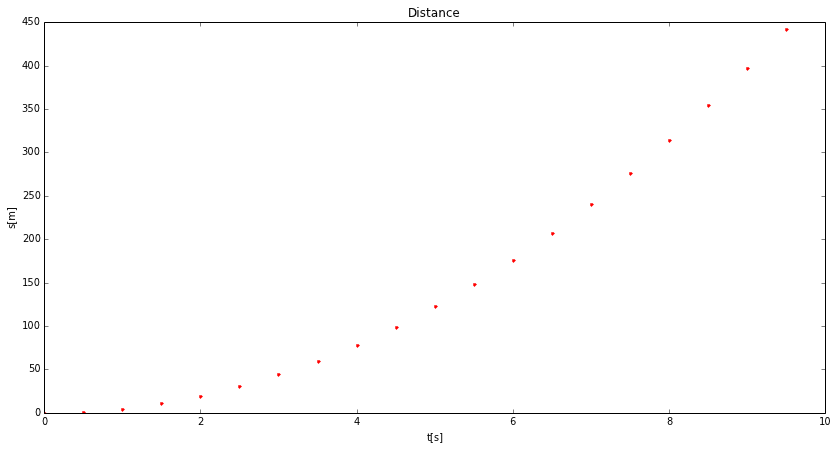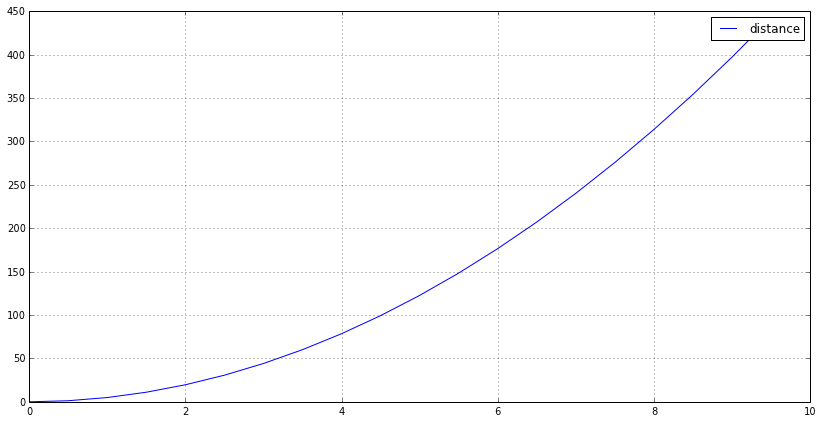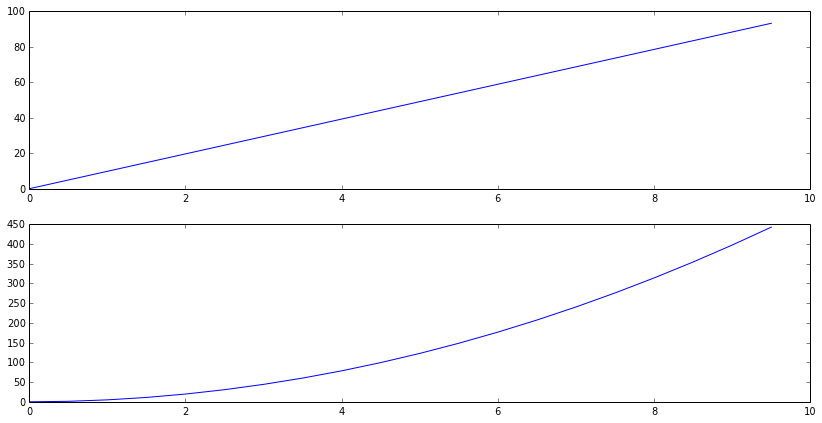## SIGNAL PROCESSING Laboratory #2

### Introduction to Python and PyLab environment

#### M. Kociński, P. Strumiłło, K. Kudryński, A. Borowska-Terka Medical Electronics Division Institute of Electronics

In :
import funkcje_do_lab2 as f2
f2.hide()

Out:
The raw code for this IPython notebook is by default hidden for easier reading. To toggle on/off the raw code, click here.

PURPOSE:

To get acquainted with basic data visualization techniques and defining functions in Python.

1. In this exercise we will use different NumPy and PyLab methods and functions
2. In an interactive mode in the Editor of Canopy type the following sequence of commands:

dt=0.5

t=np.arange(0,10,dt) # time scale
a=9.81 # acceleration
v=at # velocity
s=(a
t**2)/2. # distance

plt.plot(t,v,'.') #plot distance in discrete time instances

plt.title('Velocity')
plt.xlabel('t[s]')
plt.ylabel('v[m/s]')

plt.figure(2)
plt.plot (t,s,'.r') #plot distance in discrete time instances
plt.title('Distance')
plt.xlabel('t[s]')
plt.ylabel('s[m]')

plt.figure(3),plt.plot (t,s,label='distance') # interpolated distance
plt.legend()
plt.grid()
plt.show()

EXPECTED RESULTS:

In :
%pylab inline
pylab.rcParams['figure.figsize'] = (14.0, 7.0)
f2.plotting()

Populating the interactive namespace from numpy and matplotlibBy selecting icon [floppy disc] displayed at the top of the figure windows save (in your defined directory D:/Biomed2014_1) the displayed plots in the jpg image file format.

3.Enter the subplot commands (consult subplot? or help(subplot) commands):

figure()
subplot(2,1,1), plot (t,v) # upper plot
subplot(2,1,2), plot (t,s) # lower plot

EXPECTED RESULTS:

In :
figure()
subplot(2,1,1), plot (f2.t,f2.v)	# upper plot
subplot(2,1,2), plot (f2.t,f2.s)	# lower plot

Out:
(<matplotlib.axes.AxesSubplot at 0x75d6e30>,
[<matplotlib.lines.Line2D at 0x7659410>])4.Create a function motion(a,t) which returns the final velocity v and distance s of a body moving with acceleration a, after time t. Use the Canopy integrated editor to define and type in your function. Save the defined function under the name my_functions.py where all functions you write are stored. Now you can import your library of functions by using the command:

from my_functions import *

and then run the motion function for:
a = 5, t = 10,
a = 1, t = 5.

EXPECTED RESULTS:

In :
from my_functions import *
print 'The result of the function motion(a,t) for a = 5, t = 10:', motion(5,10)
print 'The result of the function motion(a,t) for a = 1, t = 5:', motion(1,5)

The result of the function motion(a,t) for a = 5, t = 10: (50, 250.0)
The result of the function motion(a,t) for a = 1, t = 5: (5, 12.5)



5.Write and test a new function my_sign(x) that checks, whether variable x is positive, negative or zero by printing appropriate texts, i.e. ‘positive’, ‘negative’, ‘zero’.

EXPECTED RESULTS:

In :
print 'The result of the function my_sign(x) for x = -10:', my_sign(-10)
print 'The result of the function my_sign(x) for x = 0:', my_sign(0)
print 'The result of the function my_sign(x) for x = 10:', my_sign(10)

The result of the function my_sign(x) for x = -10: negative
The result of the function my_sign(x) for x = 0: zero
The result of the function my_sign(x) for x = 10: positive



6.Write and test a new function my_stat_1d(x) which returns the minimum, average and maximum values of vector x = [1, -2, 4].

EXPECTED RESULTS:

In :
x=np.array([1, -2, 4])
print 'The result of the function my_stat_1d(x) for x = [1, -2, 4]:', my_stat_1d(x)

The result of the function my_stat_1d(x) for x = [1, -2, 4]: (-2, 1.0, 4)



7.You can also import a single function form my_functions in two possible methods:
a. from my_functions import my_stat_1d
b. from my_functions import my_stat_1d as myst

If you use method a. you invoke the my_stat_1d function e.g. by command: my_stat_1d(x)
If you use method b. you invoke the my_stat_1d function e.g. by command: myst(x)

In []: